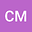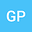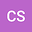loading page

Stability and flip bifurcation of a three dimensional exponential system of difference equations
•••• Chrysoula Mylona,
• Garyfalos Papaschinopoulos,
• Christos Schinas
Chrysoula Mylona
Democritus University of Thrace School of Engineering
Author ProfileGaryfalos Papaschinopoulos
Democritus University of Thrace School of Engineering
Author ProfileChristos Schinas
Democritus University of Thrace School of Engineering
Author Profile## Abstract

In this paper, we study the stability of the zero equilibrium and the occurrence of flip bifurcation on the following system of difference equations: $x_{n+1} =a_1\frac{y_n}{b_1+y_n} +c_1\frac{x_ne^{k_1-d_1x_n}}{1+e^{k_1-d_1x_n}},$\\ $y_{n+1} =a_2\frac{z_n}{b_2+z_n} +c_2\frac{y_ne^{k_2-d_2y_n}}{1+e^{k_2-d_2y_n}},$\\ $z_{n+1} =a_3\frac{x_n}{b_3+x_n} +c_3\frac{z_ne^{k_3-d_3z_n}}{1+e^{k_3-d_3z_n}}$ where $a_i$, $b_i$, $c_i$, $d_i$, $k_i$, for $i=1,2,3$, are real constants and the initial values $x_0$, $y_0$ and $z_0$ are real numbers. We study the stability of this system in the special case when one of the eigenvalues is equal to -1 and the remaining eigenvalues have absolute value less than 1, using center manifold theory.

#### Peer review status:Published

19 Oct 2020Submitted to Mathematical Methods in the Applied Sciences
20 Oct 2020Submission Checks Completed
20 Oct 2020Assigned to Editor
24 Oct 2020Review(s) Completed, Editorial Evaluation Pending
25 Oct 2020Editorial Decision: Revise Major
28 Oct 20201st Revision Received
28 Oct 2020Submission Checks Completed
28 Oct 2020Assigned to Editor
28 Oct 2020Review(s) Completed, Editorial Evaluation Pending
29 Oct 2020Editorial Decision: Accept
17 Nov 2020Published in Mathematical Methods in the Applied Sciences. 10.1002/mma.7031